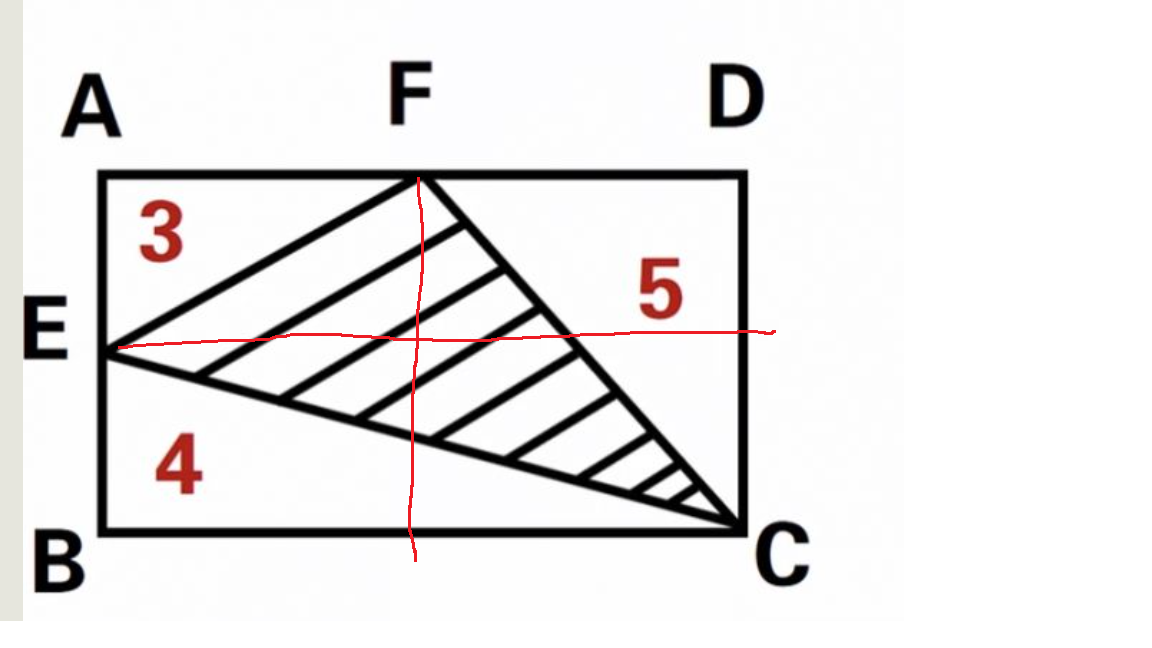# my tryDivide the rectangle in the above way, to arrive at 4 smaller rectangles. The whole rectangle area is S. Let the lower right small rectangle area be X.

S = 2*3+2*5+2*4-X = 6+10+8-X

On the other hand,  the lower right small rectangle is proportional to other areas in this way:

X/10 = 8/S

Solve to get: X=4, S=20

The shaded area is thus S-3-5-4=8.

### 加跟帖：

• 筆名：      密碼： 保持登錄狀態一個月，直到我退出登錄。
• 標題：
• 內容(可選項)： [所見即所得|預覽模式] [HTML源代碼] [如何上傳圖片] [怎樣發視頻] [如何貼音樂]